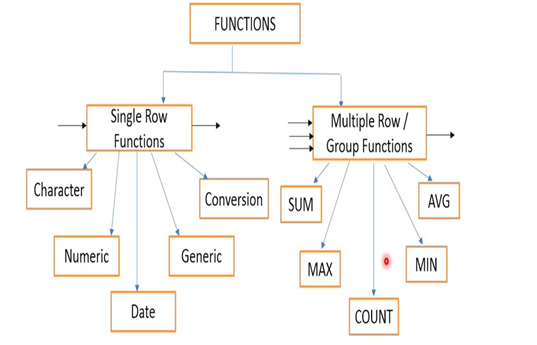# Functions in SQL

Functions are a very important topic in SQL, and they are used almost everywhere in our queries. In this blog, I will explain the concept of functions and its pre-defined types available to us.

What is a function ?

Stored piece of program that manipulates submitted data and return some value. A function is a set of SQL statements that perform a specific task. Instead of rewriting the SQL query again and again, we can simply call that function. A function accepts inputs in the form of parameters and returns a value.

SQL Server comes with a set of built-in functions that perform a variety of tasks.Where to use the functions ?

We can use functions in our queries, let’s see some examples –

• SELECT UPPER(name) FROM students

In the above example, we are using the UPPER function.

• SELECT * FROM students WHERE SUBSTRING ('SQL is FUN!’,1,3)

In the above example, we are using the SUBSTRING function.

What different types of functions are available ?

We have various types of functions available to us, but they are broadly classified as Single Row functions and Multiple Row/Group functions.The different types of functions available are as follows –

• String Functions

• Numeric Functions

• String / Number Conversion Functions

• Group Functions

• Date and Time Functions

• Date Conversion Functions

• String functions

These functions are used to do manipulation on string data.

1. LOWER( string )

All letters are changed to lowercase.

Example -  AMAR à amar

2. UPPER( string )

All letters are changed to uppercase.

Example - amar à AMAR

3. INSTR( string, find, pos, occ)

Finds string within a string.

Example - INSTR(‘Amar’, ‘ar’) à 3

INSTR(‘Amar’, ‘a’,1,2) à 3

4. LENGTH( string)

Tells length of string.

Example - LENGTH(‘Amar’) à 4

Example - LPAD(‘Amar’,10) à ‘      Amar’

Example - RPAD(‘Amar’,10) à ‘Amar      ’

7. LTRIM( string, characters)

Trims out string from left.

Example - LTRIM(‘    Amar’) à ‘Amar’

8. RTRIM( string, characters)

Trims out string from right.

Example - RTRIM(‘Amar    ’) à ‘Amar’

9. SUBSTR( string, pos, number)

Takes out string from string.

Example - SUBSTR(‘Amar’, 3) à ‘ar’

SUBTR(‘Amar’, 3, 1) à ‘a’

• Numeric functions

These functions are used to do manipulation on numeric data.

1. ABS( value)

Returns absolute value.

Example - ABS(-13) à 13

2. MOD( value, divisor)

Returns remainder of value divided by divisor.

Example - MOD(4,2) à 0

3. POWER( value, exp)

Raises power of value to its exponent.

Example - POWER(2,2) à 4

4. ROUND( value, decimal)

Rounds value to decimal places.

Example - ROUND (20.6, 0) à 21

5. CEIL ( value)

Rounds value upwards.

Example - CEIL(20.6, 0) à 21

CEIL(-20.6, 0) à 20

6. FLOOR ( value)

Rounds value downwards.

Example - FLOOR(20.6, 0) à 20

FLOOR(-20.6, 0) à 21

7. SQRT ( value)

Returns Square root of value.

Example - SQRT(36) à 6

SQRT(81) à 9

• String / Number Conversion Functions

These functions are used to convert string data to numeric data and vice-versa.

1. TO_CHAR ( value, format)

Converts value into string using format.

Example - TO_CHAR(100.11, \$999.9) à ‘\$100.1’

TO_CHAR(100.11, 999) à ‘100’

2. TO_NUMBER ( string, format)

Converts string into number using the format specified.

Example - TO_NUMBER(‘100.11’, 999.9) à 100.1

3. NANVL ( value, format)

If value NaN (Not a Number), it will replace the value.

Example - NANVL(‘Amar’, 15) à 15

NANVL(10, 15) à 10

• Group Functions

These functions are used to do manipulation on a collection or rows or multiple rows of a table.

1. Count (column)

Returns number of rows.

Example - Count (*) from orders à 140

Count (customer_id) from orders WHERE customer_id=4 à 9

2. MAX (column)

• Returns maximum value in column.

Example - MAX(marks) from students à 99

SELECT student_name from students WHERE marks = MAX(marks)

3. MIN (column)

• Returns minimum value in column.

Example - MIN (marks) from studentsà 10

SELECT student_name from students WHERE marks = MIN(marks)

4. SUM (column)

• Returns sum of column.

Example - SUM (quantity) from orders à 900

SELECT SUM(quantity) from orders WHERE customer_id= 7

5. AVG (column)

• Finds average of column

Example - AVG (rent) à \$1300

SELECT AVR(rent) from country WHERE city_id= 1

• Date and Time Functions

These functions are used to do manipulation on date and time data.

1. CURRDATE() / CURRTIME() / SYSDATE() / NOW()

Returns the current date or time.

Adds days, months and years, respectively.

3. MONTHS_BETWEEN(date1, date2)

Tells the number of months between date1 and date2.

Example - MONTHS_BETWEEN( TO_DATE ('2003/08/02', 'yyyy/mm/dd’), TO_DATE ('2003/06/02’, yyyy/mm/dd’)) à 2

4. SYSTIMESTAMP() / CURRTIMESTAMP() / TIMESTAMP ()

Returns current date and time as a timestamp.

• Date Conversion Functions

These functions are used to convert string data to date-time data and vice-versa.

1. TO_CHAR ( date, format)

Converts date into string using format.

Example -  TO_CHAR(now(), ‘dd-mm-yyyy’) à 16-8-2014

2. TO_DATE ( string, format)

Converts string into date using format.

Example - TO_DATE ('2014/08/16', 'yyyy/mm/dd')

Prev NextPARTH
DOJ:  06 Oct 2020
Topic Replies (0)
You might also like

### Not sure what course is right for you?

Choose the right course for you.
Get the help of our experts and find a course that best suits your needs.

Let`s Connect